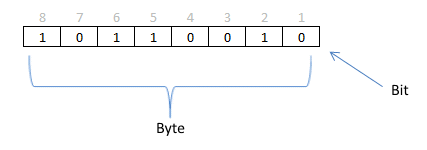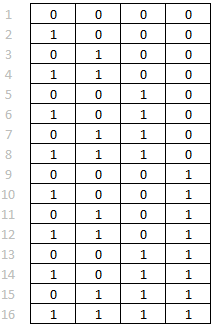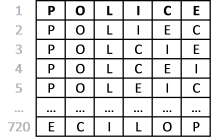Author: Oscar Cronquist Article last updated on January 21, 2023

I discussed the difference between permutations and combinations in my last post, today I want to talk about two kinds of permutations, with repetition and without repetition.

## 1. Permutations with repetition

I explained in my last post that phone numbers are permutations because the order is important. But phone numbers may also contain duplicate numbers or repeated numbers like 11 234, here number 1 is repeated. A digit in a phone number has 10 different values, 0 to 9. A five digit phone number has 10x10x10x10x10 or 10^5 equals 100 000 permutations.

Another example with repetitive numbers are bits and bytes. A bit is a single binary number like 0 or 1. A byte is a sequence of bits and eight bits equal one byte. A byte contains 256 different permutations and repetition is allowed.Here is how you calculate the number of permutations. There are two different values 0 and 1 (binary) and a byte has 8 binary values. 2x2x2x2x2x2x2x2 or 2^8 equals 256 permutations.

Did you know? The UTF-8 is a character encoding scheme using 8 bits to encode all possible characters, it is the most used encoding system on world wide web today.

## 2. Constructing a permutations array with binary valuesExcelxor showed us how to build a permutations array using only excel functions, in one of his blog posts.

The picture to the left is an array made by excelxor's formula, this example uses four cells or 4 bits. 2x2x2x2 or 2^4 equals 16 permutations.

With this type of array excelxor finds which numbers add up to a total.

Array formula:

=MOD(INT(( ROW( INDIRECT("1:"&2^ROWS(\$A\$2:\$A\$5)))-1 )/2^( TRANSPOSE( ROW( INDIRECT("1:"&ROWS(\$A\$2:\$A\$5))))-1 )), 2)

I have made a blog post a few years ago how to calculate permutations with repetition with a custom function.

## 3. Explaining array formula

#### Step 1 - Calculate number of rows

The ROWS function returns the number of rows in a cell range.

ROWS(\$A\$2:\$A\$5)

returns 4.

#### Step 2 - Concatenate characters

The ampersand character concatenates strings and numbers.

"1:"&ROWS(\$A\$2:\$A\$5)

becomes

"1:"&4

and returns  "1:4"

#### Step 3 - Create a cell reference

The INDIRECT function returns the reference specified by a text string.

INDIRECT("1:"&ROWS(\$A\$2:\$A\$5))

becomes

INDIRECT("1:4")

and returns cell reference 1:4

#### Step 4 - Return row numbers from cell reference

The ROW function returns the row number from a cell reference.

ROW( INDIRECT("1:"&ROWS(\$A\$2:\$A\$5)))

becomes

ROW( 1:4)

and returns {1; 2; 3; 4}. These numbers are in a vertical range.

#### Step 5 - Transpose numbers

The TRANSPOSE function converts a vertical range to a horizontal range or vice versa.

TRANSPOSE( ROW( INDIRECT("1:"&ROWS(\$A\$2:\$A\$5))))

becomes

TRANSPOSE( {1; 2; 3; 4})

and returns {1, 2, 3, 4}.

#### Step 6 - Subtract with 1

( TRANSPOSE( ROW( INDIRECT("1:"&ROWS(\$A\$2:\$A\$5))))-1 )

becomes

{1, 2, 3, 4} - 1

and returns {0, 1, 2, 3}

#### Step 7 - Remove decimals from numbers

The INT function removes the decimal part from positive numbers and returns the whole number (integer) except negative values are rounded down to the nearest integer.

INT(( ROW( INDIRECT("1:"&2^ROWS(\$A\$2:\$A\$5)))-1 )/2^( TRANSPOSE( ROW( INDIRECT("1:"&ROWS(\$A\$2:\$A\$5))))-1 ))

becomes

INT(( ROW( INDIRECT("1:"&2^ROWS(\$A\$2:\$A\$5)))-1 )/2^({0, 1, 2, 3}))

becomes

INT(( ROW( INDIRECT("1:"&2^4))-1 )/2^({0, 1, 2, 3}))

becomes

INT(( ROW( INDIRECT("1:"&16))-1 )/2^({0, 1, 2, 3}))

becomes

INT(( {1; 2; 3; 4; 5; 6; 7; 8; 9; 10; 11; 12; 13; 14; 15; 16}-1 )/2^({0, 1, 2, 3}))

becomes

INT({0; 1; 2; 3; 4; 5; 6; 7; 8; 9; 10; 11; 12; 13; 14; 15; 16}/2^({0, 1, 2, 3}))

becomes

INT({0; 0.5; 1; 1.5; 2; 2.5; 3; 3.5; 4; 4.5; 5; 5.5; 6; 6.5; 7; 7.5}^({0, 1, 2, 3}))

becomes

INT({0, 0, 0, 0; 1, 0.5, 0.25, 0.125; 2, 1, 0.5, 0.25; 3, 1.5, 0.75, 0.375; 4, 2, 1, 0.5; 5, 2.5, 1.25, 0.625; 6, 3, 1.5, 0.75; 7, 3.5, 1.75, 0.875; 8, 4, 2, 1; 9, 4.5, 2.25, 1.125; 10, 5, 2.5, 1.25; 11, 5.5, 2.75, 1.375; 12, 6, 3, 1.5; 13, 6.5, 3.25, 1.625; 14, 7, 3.5, 1.75; 15, 7.5, 3.75, 1.875})

and returns {0, 0, 0, 0; 1, 0, 0, 0; 2, 1, 0, 0; 3, 1, 0, 0; 4, 2, 1, 0; 5, 2, 1, 0; 6, 3, 1, 0; 7, 3, 1, 0; 8, 4, 2, 1; 9, 4, 2, 1; 10, 5, 2, 1; 11, 5, 2, 1; 12, 6, 3, 1; 13, 6, 3, 1; 14, 7, 3, 1; 15, 7, 3, 1}.

#### Step 8 - Create binary array

The MOD function returns the remainder after a number is divided by divisor.

MOD(INT(( ROW( INDIRECT("1:"&2^ROWS(\$A\$2:\$A\$5)))-1 )/2^( TRANSPOSE( ROW( INDIRECT("1:"&ROWS(\$A\$2:\$A\$5))))-1 )), 2)

becomes

MOD({0, 0, 0, 0; 1, 0, 0, 0; 2, 1, 0, 0; 3, 1, 0, 0; 4, 2, 1, 0; 5, 2, 1, 0; 6, 3, 1, 0; 7, 3, 1, 0; 8, 4, 2, 1; 9, 4, 2, 1; 10, 5, 2, 1; 11, 5, 2, 1; 12, 6, 3, 1; 13, 6, 3, 1; 14, 7, 3, 1; 15, 7, 3, 1} , 2)

and returns {0, 0, 0, 0; 1, 0, 0, 0; 0, 1, 0, 0; 1, 1, 0, 0; 0, 0, 1, 0; 1, 0, 1, 0; 0, 1, 1, 0; 1, 1, 1, 0; 0, 0, 0, 1; 1, 0, 0, 1; 0, 1, 0, 1; 1, 1, 0, 1; 0, 0, 1, 1; 1, 0, 1, 1; 0, 1, 1, 1; 1, 1, 1, 1}.

## 4. Permutations without repetitionImagine constructing an anagram of a word "police". You are allowed to rearrange the letters but you can´t repeat a letter. See picture to the right.

Another example is how many times can you rearrange 6 people around a table? Most people will get annoyed if you ask them to change seat more than once but in this example you can rearrange as many times as you like. How many permutations are there? You can´t have a person on two chairs at the same time, repetition is not allowed.

Here is a link to a udf I made a few years ago: Excel udf: List permutations without repetition

Tip! Use the PERMUT function to calculate permutations. The word police has 6 letters, how many permutations without repetition are there if you choose 6 out of 6 letters?
=PERMUT(6,6) equals 720 permutations.